# How to Find Duplicate Characters on String - Java Programming Problems

Today's programming exercise is to write a program to find repeated characters in a String. For example, if given input to your program is "Java", it should print all duplicates characters, i.e. characters appear more than once in String and their count e.g. a = 2 because character 'a' has appeared twice in String "Java". This is also a very popular coding question on the various level of Java interviews and written test, where you need to write code. On difficulty level, this question is at par with prime numbers or Fibonacci series. I personally like this exercise because it gives beginners an opportunity to familiar with the concept of Map data structure, which allows you store mappings in the form of key and value. Since Map is heavily used in any enterprise Java application, good knowledge of this data structure is highly desirable among any level of Java programmers.

By the way, there are a couple of variants of this problem, which you may want to look before going for an interview. Sometimes an interviewer will ask you to read a file and print all duplicate characters and their count, core logic will remain same, all you need to do is demonstrate how much you know about File IO in Java e.g. streaming file if it's very large rather than reading the whole file in memory.

## Java Program to find Repeated Characters of String

The standard way to solve this problem is to get the character array from String, iterate through that and build a Map with character and their count. Then iterate through that Map and print characters which have appeared more than once. So you actually need two loops to do the job, the first loop to build the map and second loop to print characters and counts.

If you look at below example, there is only one static method called printDuplicateCharacters(), which does both this job. We first got the character array from String by calling toCharArray().

Next we are using HashMap to store characters and their count. We use containsKey() method to check if key, which is a character already exists or not, if already exists we get the old count from HashMap by calling get() method and store it back after incrementing it by 1.Once we build our Map with each character and count, next task is to loop through Map and check each entry, if count, which is the value of Entry is greater than 1, then that character has occurred more than once. You can now print duplicate characters or do whatever you want with them.

```import java.util.HashMap;
import java.util.Map;
import java.util.Scanner;
import java.util.Set;

/**
* Java Program to find duplicate characters in String.
*
*
* @author http://java67.blogspot.com
*/
public class FindDuplicateCharacters{

public static void main(String args[]) {
printDuplicateCharacters("Programming");
printDuplicateCharacters("Combination");
printDuplicateCharacters("Java");
}

/*
* Find all duplicate characters in a String and print each of them.
*/
public static void printDuplicateCharacters(String word) {
char[] characters = word.toCharArray();

// build HashMap with character and number of times they appear in String
Map<Character, Integer> charMap = new HashMap<Character, Integer>();
for (Character ch : characters) {
if (charMap.containsKey(ch)) {
charMap.put(ch, charMap.get(ch) + 1);
} else {
charMap.put(ch, 1);
}
}

// Iterate through HashMap to print all duplicate characters of String
Set<Map.Entry<Character, Integer>> entrySet = charMap.entrySet();
System.out.printf("List of duplicate characters in String '%s' %n", word);
for (Map.Entry<Character, Integer> entry : entrySet) {
if (entry.getValue() > 1) {
System.out.printf("%s : %d %n", entry.getKey(), entry.getValue());
}
}
}

}

Output
List of duplicate characters in String 'Programming'
g : 2
r : 2
m : 2
List of duplicate characters in String 'Combination'
n : 2
o : 2
i : 2
List of duplicate characters in String 'Java'```

That's all on how to find duplicate characters in a String. Next time if this question is asked to you in a programming job interview, you can confidently write a solution and can explain them. Remember this question is also asked as write a Java program to find repeated characters of a given String, so don't get confused yourself in wording, the algorithm will remain same.

Further Learning
The Coding Interview Bootcamp: Algorithms + Data Structures
Data Structures and Algorithms: Deep Dive Using Java
Algorithms and Data Structures - Part 1 and 2

1.How do you do it without using any additional data structure.

1.import java.io.*;
public class Str
{
public static void main(String arg[])throws Exception
{
String res="";
while(a.length()>0)
{
int c=0;
for(int j=0;j<a.length();j++)
{
if(a.charAt(0)==a.charAt(j))
c=c+1;
}
res=res+a.charAt(0)+"="+c+"\n";
String k[]=a.split(a.charAt(0)+"");
a=new String("");
for(int i=0;i<k.length;i++)
a=a+k[i];
}
System.out.println(res);
}
}

2.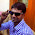Thanks its very useful to me..

3.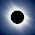Good solution, but you can see without using data structure time complexity of your solution grows from O(n) to O(n^2). This is actually good learning point, choice of good data structure improves your algorithm.

4.Thanks "Anonymous", very helpful stuff for searching with minimal resources.

5.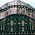package StringPac;

import java.util.Scanner;

/**
* @author Tarique
* How to Print duplicate characters from String?
*/
public class duplicateChar {

/**
* @param args
*/
public static void main(String[] args)
{

//String str = "Nitin";

Scanner sc = new Scanner(System.in);

String str = sc.next();

char d = 0;
int count = 0;
/* for(int i=0;i<str.length();i++)
{

char c = str.charAt(i);
System.out.println(c);
}*/
for (int i = 0; i < str.length(); i++)
{

for (int j = i+1; j < str.length(); j++)
{

if (str.charAt(i)==str.charAt(j))
{
if(d!=str.charAt(i))
{
count++;

d = str.charAt(i);

System.out.println("Duplicate Charaacter is "+d);
break;
}

}
}
}
System.out.println("Number of duplicate character is "+count);
}

}

2.Why is the 'a' of Java not occuring twice?

1.@Anonymous, It actually does print 'a' twice for 'Java', its just that I missed when I copied output from Eclipse. You can try running program in your machine, it will print.

List of duplicate characters in String 'Java'
a : 2

3.How to add two arrays A={3,5,2,1} and B={6,8,9,2,4} index by index into third array C={4,5,8,9,6} answer this please..

1.@Vekatesh, if all array of same length, you can use a for loop to do this job. Just iterate over both array, take each element from same index, add them and store into third array.

2.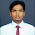///please refer below code to copy one arrays into other ,many approaches is for this but i have implemented only two
package com.java8.demo;

import java.lang.reflect.Array;
import java.util.ArrayList;
import java.util.Arrays;
import java.util.List;
import org.apache.commons.lang.ArrayUtils;

public class MergeArrays {

public static void main(String[] args) {
// TODO Auto-generated method stub
String a[]={"a","b","c","r","g","f"};
String b[]={"d","e"};
//method 1
Object[] combined = ArrayUtils.addAll(a, b);
System.out.println("combined arrays are:"+Arrays.toString(combined));
//method 2
List ls=new ArrayList<>(Arrays.asList(a));
Object o[]=ls.toArray();
System.out.println("Merged arrays Is:"+Arrays.toString(o));
//for sorting the arrays
Arrays.sort(o);
System.out.println("sort the merged ayyars:"+Arrays.toString(o));
int d=Arrays.binarySearch(o, "b");
System.out.println("Searched Key's index is:"+Arrays.binarySearch(o, "k")+d);

}

}

4.You can also find using a single loop.

public static boolean checkRepeatingCharactersFromMap(String s1) {
boolean repeat = false;
if (s1 != null && s1.length() > 0) {
char[] s1Array = s1.toCharArray();

Set set = new TreeSet();
Set repeatChar = new TreeSet();

for (char c1: s1Array) {
// System.out.print(c1 + " "); //if you want to print each occurance of the repeating character
repeat = true;
// return true; //end the loop if you don't want to cache the repeating characters
}
}

System.out.print(repeatChar);
}

return repeat;
}

1.@Genius, it will work but it will not print duplicates in the order they appear in original String. Nevertheless, if that's not stated, you can use it. Replacing TreeMap with LinkedHashMap will print the repeated character in the same order they appear in given input.

5.String s = "javaqjjcxcdf";
char[] charArray = s.toCharArray();

Map map = new HashMap();
/*for (Character character : charArray) {
if (map.containsKey(character)) {
map.put(character, map.get(character) + 1);
} else {
map.put(character, 1);
}
}*/

for (Character character : charArray) {
map.put(character, map.get(character) != null?map.get(character)+1:1);
}
System.out.println(map);

1.@Vikas, good choice of using ternary operator, much cleaner.

2.Just one changed need as the type of map should like Map map = new HashMap();

All good, good to see example with one loop itself.

6.Python code:

duplicate=[]
first_time=[]

a = ""Hello world"

for i in a:
if i not in first_time:
first_time.append(i)
else:
duplicate.append(i)

print "".join(duplicate)

1.@Anonymous, Thanks for providing Python solution, neat.

7.l1=list('Java')
l2=set(l1)
[l1.remove(x) for x in l2]
print l1

1.Hello @Anonymous, Nice solution, is it Groovy, Scala or Ruby?

8.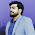@Javin: How about my solution?
My solution even prints in alphabetical order. Very much memory efficient. If the question is only to find those characters which occur more than once, then my solution runs perfectly fine.

import java.util.*;
import java.io.*;

class Ideone
{
public static void main (String[] args) throws IOException
{

String s = br.readLine();
int len = s.length();

int[] charCount = new int;

for(int i = 0; i=65 && c<=90)
c=(char)(c+32);
if(c>=97 && c<=122)
charCount[c-97]+=1;
}

for(int i = 0; i<26; i++){
if(charCount[i]>1)
System.out.println((char)(i+97) + " : " + charCount[i]);
}
}
}

1.what is variable c ? atleast put the code without compilation errors

9.package Strings;

import java.util.ArrayList;
import java.util.List;

public class PrintDuplicateStrings {
public static void main(String[] args) {
String s = "hello world";
char[] chars = s.toCharArray();
int i,j = 0;
List finalList = new ArrayList();

for(i=0;i<chars.length;i++){
for(j=i+1;j<chars.length;j++){
if(!finalList.contains(String.valueOf(chars[i])) && chars[i]==chars[j]){
}
}
}

System.out.println("-- "+finalList);
}
}

1.You can avoid inner loop by adding a one more condition before inner loop, that avoids inner loop if a repeated character occurs.

public static void printDuplicateCharsInString(String str) {
if(str==null && str.length() <=0 ) {
return;
}
int length= str.length();
List list= new ArrayList<>();
char ch;
int counter;
for(int i=0; i<length ;i++ ) {
ch=str.toLowerCase().charAt(i);
counter=1;
if( !list.contains(ch) && ch !=' ') {
for(int j=i+1;j<length; j++) {
if(ch== str.charAt(j)) {
counter++;
//break;
}
}
System.out.println("Character: '"+ch +"' occurs "+counter+" times");
}
}
System.out.println("Duplicate Chars: "+list.toString());
}

10.//Program to print duplicate characters in string
/* package whatever; // don't place package name! */

import java.util.*;
import java.lang.*;
import java.io.*;

/* Name of the class has to be "Main" only if the class is public. */
class Ideone
{
static boolean find(char[] update,char ch){
for(int i=0;i<update.length;i++)
if(update[i]==ch)
return false;
return true;
}
static void solution(String str){
char[] ar=str.toCharArray();
char[] update=new char[ar.length];
int i=0,z=0;
while(i<ar.length-1){
int j=i+1;
while(j<ar.length){
if(find(update,ar[j])&&ar[i]==ar[j]){
update[z++]=ar[i];
System.out.print(ar[i]+" ");
break;
}
j++;
}
i++;
}
}
public static void main (String[] args) throws java.lang.Exception
{
// your code goes here
Scanner scan=new Scanner(System.in);
String str=scan.nextLine();
solution(str);
}
}

11.i am getting null pointer exception how to solve it

12.I need to know how you print a character occurrence count Eg: Strins s = "1111223331123444" so o/p should be 14223312213143

13.package Arrys;

import java.util.ArrayList;
import java.util.Collection;
import java.util.Collections;
import java.util.HashMap;
import java.util.Hashtable;
import java.util.List;

public class TwoStringPrograme {

public static void main(String[] args) {
String a="aaaxbbcccbaaasss";
char ch;
List cha=new ArrayList();
int count1;
char a1[]=a.toCharArray();
for(int i=0;i<a1.length;i++)
{
count1=0;
ch=a.charAt(i);

if(!cha.contains(ch))
{
for(int j=0;j<a1.length;j++)
{

if(ch==a1[j])
{
count1++;

}

}
System.out.println(ch+"--"+count1);
}
}

} }

14.// without using any buildin function

public static void main(String[] args) {
char[] array = "aabsbdcbdgratsbdbcfdgs".toCharArray();
char[][] countArr = new char[array.length];
int lastIndex = 0;
for (char c : array) {
int foundIndex = -1;
for (int i = 0; i < lastIndex; i++) {
if (countArr[i] == c) {
foundIndex = i;
break;
}
}
if (foundIndex >= 0) {
int a = countArr[foundIndex];
countArr[foundIndex] = (char) ++a;
} else {
countArr[lastIndex] = c;
countArr[lastIndex] = '1';
lastIndex++;
}
}
for (int i = 0; i < lastIndex; i++) {
System.out.println(countArr[i] + " " + countArr[i]);
}
}

15.public void printDups(String str) {

if(str != null && str.length() > 0) {
char [] dupArr = str.toCharArray();

System.out.println("Dup Array ");
String dups ="";
for(int i=0; ii; j--){
if (dupArr[i] == dupArr[j]) {
dups = (dups+dupArr[i]).trim();
System.out.print(dupArr[i]);
}
}
}

}
}

16.Instead of clumsy solutions above, just loop the string once from left to right so to speak and at every character check if it also exists on the right hand side yet left to loop. A one liner and much easier to read. Work smarter, not harder!

17.#python Code
import sys

myStr='java'
#try with javaav

first_time=[]
duplicate=[]

#Empty String
if len(myStr)<1:
print "empty string"
sys.exit()

#iterating over the string
for i in myStr:
if i not in first_time:
first_time.append(i)
else:
if i not in duplicate:
duplicate.append(i)
print "".join(duplicate)

output: a
output 2nd test case: av
Credits- above anonymous coder

18.class Practise3

{

public static void main(String[] args) throws ArrayIndexOutOfBoundsException
{

for(int i=0;i<args.length;i++)
{
System.out.println("Enter the string :" + args[i]);
String s = (String)args[i];
System.out.println(s);
try
{
for( int k =0; k<s.length();k++)
{
for(int j = k+1; j!=s.length();j++)
{
char var = s.charAt(k);
if(var == s.charAt(j))
{
System.out.println("duplicate character is : " + s.charAt(j));
}
else
{
System.out.println(" no duplicates found" + ".." + s.charAt(k));
}
}
}
}
catch (Exception e)
{
System.out.println("no");
}

}

}
}
I just tried using String class and its methods

19.Why not recursion? It's powerful and logical.

public class StringDuplicate {
//search from the beginning of the string
public static void printDupes(String string) {
//specify exit case, when we finish the whole string
if(string.length() == 0) return;
//find the string in which the character you're searching for is dropped
String substring = string.substring(1);
//if we found that character on the substring, we sysout that b**ch
if(substring.indexOf(string.charAt(0)) > 0) {
System.out.println(string.charAt(0));
}
//we let the recursion go to the shorter string...
//by the process of induction, this will always terminate ;)
printDupes(substring);
}
public static void main(String[] args) {
printDupes(args);
}
}

20.Why not recursion? Less storage, sleek, unique, and cool! :)
public class StringDuplicate {
public static void printDupes(String string) {
//exit condition
if(string.length() == 0) return;
//find the substring in which to see if the first character is in
String substring = string.substring(1);
//what you do if you find it? print it!
if(substring.indexOf(string.charAt(0)) > 0) {
System.out.println(string.charAt(0));
}
//recusion on that b*tch
printDupes(substring);
}
public static void main(String args[]) {
printDupes(args); //utilize command line arguments for general cases
}
}

1.@artsArt, yes, you can use recursion, no problem with that, but your solution will only print the duplicate characters, not their count. How can you improve the solution to print the count of duplicate character?

21.checks for words separated by space, removers symbols too.

def removechar(dup):
symbol = "~`!@#\$%^&*()_-+={}[]:>;',</?*-+"
for i in dup:
if i in symbol or i is ' ':
dup.remove(i)
return dup
def duplicate(str):
l = []
dup = []
str = list(str)
for i in str:
try:
j = list(i)
for k in j:
if k not in l:
l.append(k)
else:
dup.append(k)
except:
print "No Duplicate"
if len(dup) == '0':
print "No Dupliates"
else:
d = []
final = dup
for i in dup:
if i not in d:
d.append(i)
print "Total numbe of duplicates:",len(removechar(d)),"\nThey are:",','.join(removechar(d))
if __name__ == '__main__':
duplicate(raw_input("Enter the string"))

22.public static void printDuplicatechar(String s) {

char[] arr = s.toCharArray();

List list=new ArrayList<>();

for (int i = 0; i < arr.length; i++) {
int h = 0;
for (int j = 0; j < arr.length; j++) {
if (arr[i] == arr[j]) {

h++;
if (h >= 2) {

}
}
}
}
list.stream().distinct().forEach(e->System.out.println(e));
}

23.Map map = Arrays.stream(s.split("")).collect(Collectors.groupingBy(c -> c , Collectors.counting()));
List duplicates = map.entrySet().stream().filter(entry -> entry.getValue() > 1).map(k -> k.getKey()).collect(Collectors.toList());

24.package logic;
public class DuplicateChar {
public static void findDuplicateCharacters(String input1)
{
int i;

char[] a=input1.toCharArray();
int h[]=new int;
for(i=0;i64&&a[i]<95)
{
h[a[i]-65]++;
}
if(a[i]>95&&a[i]<123)
{
h[a[i]-97]++;
}
}
for(i=0;i64&&a[i]<95)
{
if(h[a[i]-65]>1)
System.out.println(a[i]+" "+h[a[i]-65]);
h[a[i]-65]=0;
}

if(a[i]>95&&a[i]<123)
{
if(h[a[i]-97]>1)
System.out.println(a[i]+" "+h[a[i]-97]);
h[a[i]-97]=0;
}
}
}
public static void main(String args[])
{

findDuplicateCharacters("programming");
}
}

25.public class Duplicatechar {
public static void main(String[] args) {
String s ="java";
char x;
int i;
int c=0;
String str2=s.substring(1);

for(i=0;i<s.length();i++)
{
c=0;
x=s.charAt(i);
for(int j=0;j<str2.length();j++)
{
char y=str2.charAt(j);
if(x==y)
{
c++;

if(c==2)
System.out.println("the duplicate char "+x);
}
}

}

}

}
I have one mistake it print for me twice the comment that the duplicate character is a .. can anyone help me .. thanks

26.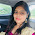public class RemoveDup
{
public static void main(String args[])
{
String s;
int i;
Scanner sc=new Scanner(System.in);
System.out.println("Enter any String");
s=sc.next();
char[] chars= s.toCharArray();
Set charSet = new LinkedHashSet();
for (char c : chars)
{
}
StringBuilder sb = new StringBuilder();//to buffer characters
for (Character character : charSet)
{
sb.append(character);
}
System.out.println(sb.toString());
}
}

27.#include
#include

void main()
{

char s,ch;
int i,j,n,flag=0;

printf("Enter\n");
scanf_s("%s",s,8);

n=strlen(s);

printf("%d\n", n);

for(i=0;i=2)
{

printf("%c\n", ch);

}
else
{
printf("No\n");

}

}

28.#include
#include
#include

using namespace std;

struct node
{
char ch;
int count;
struct node *next;
};
void main ()
{
node * Head = NULL;
node * crawl = Head;
char input;
memset(input,0,100);
printf("Enter the string :- \n");
scanf("%[^\n]s",&input);

if(input == NULL)
{
printf("NULL String!!!");
getch();
return;
}
int nLen = strlen(input);
if(nLen <= 1)
{
printf("Invalid String!!!");
getch();
return;
}

for(int n = 0; n 0)
{
{
Head = new node();
}
else
{
while (crawl->next != NULL && crawl->ch != ref)
{
crawl= crawl->next;
}

if(crawl->next == NULL)
{
if(crawl->ch != ref)
{
crawl->next = new node();
crawl = crawl->next;
crawl->next = NULL;
crawl->ch = ref;
crawl->count = nCount;
}
}
}
}

}

{

delete crawl;
}
}

29.#include
#include
#include

using namespace std;

struct node
{
char ch;
int count;
struct node *next;
};
void main ()
{
node * Head = NULL;
node * crawl = Head;
char input;
memset(input,0,100);
printf("Enter the string :- \n");
scanf("%[^\n]s",&input);

if(input == NULL)
{
printf("NULL String!!!");
getch();
return;
}
int nLen = strlen(input);
if(nLen <= 1)
{
printf("Invalid String!!!");
getch();
return;
}

for(int n = 0; n 0)
{
{
Head = new node();
}
else
{
while (crawl->next != NULL && crawl->ch != ref)
{
crawl= crawl->next;
}

if(crawl->next == NULL)
{
if(crawl->ch != ref)
{
crawl->next = new node();
crawl = crawl->next;
crawl->next = NULL;
crawl->ch = ref;
crawl->count = nCount;
}
}
}
}

}

{

delete crawl;
}
}

30.See following solution with O(n) complexity.

public static void FindDuplicateCharacters(String str){

if(str == null || str.length() == 0){
System.out.println("Invalid String");
return;
}

int[] counter = new int;
for(char c : str.toCharArray()){
counter[c]++;
}

for(int i = 0 ; i < str.length(); i++){
char c = str.charAt(i);
if(counter[c] > 1){
System.out.println(c);
counter[c] = 1;
}
}
}

31.a=raw_input("Enter The String")

print set([x for x in a if a.count(x)>1])

In Python

32.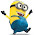Scanner sc = new Scanner(System.in);
String inputString = sc.next();
System.out.println("Your String ::: " + inputString);

if(null != inputString && inputString.length() > 0) {
for(int i = 0; i < inputString.length(); i++) {
int j = i;
while(j > 0) {
if(inputString.charAt(i) == inputString.charAt(j-1) )
System.out.println(inputString.charAt(i));
j--;
}
}
}
sc.close();

33.I will use map.put(c[i], map.getOrDefault(c[i], 1) + 1);
to avoid else condition

1.Hello Nitesh, that is good but how about getOrDefault() method, do you know from which Java version it is available?

34.i'm beginner so my solution maybe is not rational. I tried it in c++

#include
#include
using namespace std;

void main()
{
char s, *p, s_="", s1="";
cin >> s;
p=s;
while (strlen(p)>0)
{
strncpy_s(s_, p, 1);
strncpy_s(s1, s, strlen(s)-strlen(p));
if(strrchr(p, *p)!=strchr(p, *p)
&& strchr((p+strcspn(p, s_)+1), *p)==strrchr(p, *p)
&& strcspn(s1, s_)==strlen(s1) )
cout << *p << ": " << 2 << endl;
p++;
}
system("pause");
}

35.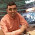import java.util.Scanner;

public class DuplicateString {

public static void main(String[] args) {
Scanner sc = new Scanner(System.in);

char[] str = sc.next().toLowerCase().toCharArray();

int[] charArray = new int;

for (int i = 0; i < str.length; i++) {
charArray[Math.abs(97-str[i])]++;
}

for (int i = 0; i < charArray.length; i++) {
if (charArray[i] > 1) {
System.out.printf("%c",Math.abs(97+i));
System.out.println();
}
}
}
}

36.#include

2

#include

3

#include

4

#include

5

6

int main() {

7

char a;

8

int i,j,has={0},l;

9

scanf("%s",a);

10

l=strlen(a);

11

for(i=0;i1)

17

{

18

printf("%c -> %d\n",i+65,has[i]);

19

}

20

}

21

22

}

23

37.private static void characterCount(String str) {
HashMap charCountMap = new HashMap<>();
for (Character ch : str.toLowerCase().toString().toCharArray()) {
Integer count = charCountMap.putIfAbsent(ch, 1);
if (null != count) {
charCountMap.put(ch, charCountMap.get(ch) + 1);
}
}
Map charMap =
charCountMap.entrySet().stream().filter(map -> map.getValue() != 1).collect(Collectors.toMap(p -> p.getKey(), p -> p.getValue()));
System.out.println(charMap);
}

38.public class RemoveDuplicatesFromString {

public static void main(String[] args) {

String input = "hello world";
String output = removeDuplicates(input);
System.out.println(output);
}

private static String removeDuplicates(String input) {
HashSet mySet = new HashSet();
String output="";

for(Character c :input.trim().replaceAll(" ", "").toCharArray())
{
if(!output.contains(c+""))
output = output+c;
}
return output;
}
}

39.import java.util.Scanner;

public class JavaApplication19 {
public static void main(String[] args) {
Scanner sc=new Scanner(System.in);
System.out.println("Program to print repeated characters in a string");
System.out.println("Enter the string");
String s1=sc.next();
System.out.println("Repeated characters in the given string is:");
for(int i=0;i=2){
System.out.print(s1.charAt(i));
}
}
}
}
System.out.println();
}
}

40.import java.util.Scanner;
public class JavaApplication19 {
public static void main(String[] args) {
Scanner sc=new Scanner(System.in);
System.out.println("Program to print repeated characters in a string");
System.out.println("Enter the string");
String s1=sc.next();
String s2="";
int flag=0;
for(int i=0;i=2){
flag=1;
s2=s2+String.valueOf(s1.charAt(i));
}
}
}
}
if(flag==1){
System.out.println("Repeated characters in the given string is:");
System.out.println(s2);
}else{
System.out.print("NULL");
}
System.out.println();
}
}

41.import java.util.List;
import java.util.ArrayList;
import java.util.Scanner;
public class S5 {
static int k=0,val=0;
public static void main(String[] args) {
Scanner sc = new Scanner(System.in);
String s1=sc.next();
int count=0;
String otherString="";
List l1= new ArrayList();
for( char i='a';i<='z';i++){

count = s1.length()-s1.replaceAll( String.valueOf(i) ,"").length();
// System.out.println(count);
if(count>1){
String character = ""+i;
otherString = otherString.concat(character);

}
}
char[] ch=otherString.toCharArray();
int[] ret = new int[l1.size()];
int j = 0;
for (Integer e : l1)
ret[j++] = e.intValue();
for(int i=0;i<ch.length;i++)
{
System.out.println(ch[i]+": "+ret[i]);
}

}
}

42.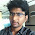public class Duplicatechar{
//How do you do it without using any additional data structure.
public static void main(String[] args)
{
String name= "javava", rev="";
for(int i=0; i<name.length(); i++)
{
int count =1;
for(int j=0; j<name.length(); j++){
if(i != j){
while(name.charAt(i)==name.charAt(j)){
count++;
break;
}
}
}
if(rev.indexOf(name.charAt(i))!=-1){
System.out.print("");
}else{
rev += String.valueOf(name.charAt(i));
System.out.println("character is ="+name.charAt(i)+" and Count is = "+count);
}
}

}
}

43.U can add counter to count if 2 or more duplicates are in that word and store it in list as well. List is optional just for easy lookup for duplicates.
Simple way:-->

import java.util.ArrayList;
import java.util.List;

public class Multy {

public static void main(String[] args){
String[] words = {"Programming","Karma","Colleague"};
FindMultiplications(words);
}
static void FindMultiplications(String words){
char[] h = words.toCharArray();
List cont = new ArrayList<>();
for (int i=0;i<words.length();i++){
for (int j=0;j<words.length();j++){
if (i==j) continue;
else if (h[i]==h[j] && !(cont.contains(h[i]))){
System.out.println("Duplicate exist "+h[i]+" "+h[j]);
}
else System.out.println("Print "+h[i]+" "+h[j]);
}
}
}
}

44.package com.stringprac;

import java.util.Scanner;

public class CharCount {

public static void main(String[] args) {

Scanner sc = new Scanner(System.in);
System.out.println("please enter a string");
String s = sc.nextLine();
int count=1;
for(int i=0; i<s.length(); i++) {
for(int j=i+1; j<s.length(); j++) {
if(s.charAt(i)==s.charAt(j)) {
count++;
System.out.println(s.charAt(i)+": "+count);
}
}
count=1;
}
}
}

45.If you want only to print duplicate characters...
Here, I'm iterating through only half of the String. Suggestions for improvement are always welcome :)

// Print duplicate characters of a String.
public static void printDuplicates(String str) {
if (str.length() == 1) {
System.out.println("No duplicate found!");
return;
}
char[] arr = str.toCharArray();
Set setOfDuplicates=new HashSet();
int length = arr.length;
List list=new ArrayList();
for (int i = 0; i < (length) / 2; i++) {
System.out.println("i= "+i);

if(list.contains(arr[i]))
else
if(list.contains(arr[length-i-1]))
else
}
System.out.println(setOfDuplicates);
}

46.//Print repetive characters from string.
public static void GetDuplicateChars()
{
string str="TestMyTesty";
for(int i=0;i-1)
Console.Write(str[i]);
}
}

1.public static void main(String[] args) {
String input = "kdsfglčjretg945ghdčfsgfjki235";
Map M = new HashMap<>();
for (char c : input.toCharArray()) {
String s = String.valueOf(c);
M.compute(s, (String key, Integer value) -> {
return value == null ? 1 : 1 + value;
});
}

System.err.println(M);
}

47.Given a string print only repeated individual
characters of the string in the order they occur

if no such characters occur print -1

input =
abcac
output =
acac

input =
ahdaa
output =
aaa

input =
ijkl
output =
-1

48.public Set getDuplicates(String string) {
Set toReturn = new LinkedHashSet<>();
if (string == null || string.isEmpty()) {
} else {
for (int i = 0; i < string.length(); i++) {
Character c = string.charAt(i);
if (string.substring(i + 1, string.length()).contains(c.toString())) {
}
}

}

}

49.This is my version of this problem
public static char duplicateCharInString(String input) {
char ch =' ';
Set nonDuplicate = new HashSet();
for(int i=0;i<input.length();i++) {
if(!nonDuplicate.contains(input.charAt(i))){
}
else {
return input.charAt(i);
}
}
return ch;
}

50.C/C++ Solution:

#include
#include

using namespace std;

void getDuplicate(char ch[]);
int main()
{
cout << "\n String Test Program";
char ch;
cout << "\nEnter input string: ";
cin >> ch;

getDuplicate(ch);

_getch();
return 0;
}

void getDuplicate(char ch[])
{
int Arr = { 0 };
for (int i = 0; i < strlen(ch); i++)
{
Arr[ch[i]] += 1;
}

for (int i = 0; i < 255; i++)
{
if (Arr[i] >= 2)
cout << char(i) << " ";
}
}

51.`` public static String printDuplicate(String s) {
int counts[] = new int;
StringBuilder res = new StringBuilder();

for (char c : s.toCharArray()) counts[c]++;

for (int i = 0; i < counts.length; i++) {
if (counts[i] > 1) res.append((char) i);
}
return res.toString();
}
``

1.Good job. did you tested it for null and empty values? though, not required here, you should always handle edge cases, now a days many companies using interview portals to check your solution which has many test cases with boundary conditions. Though this solution is all right it will not pass those edge cases.

52.Anybody knows how to find and remove duplicate words in as string with concept of hashtable and string tokenizer

1.Hello @Anonyous, check these tutorials, you may get some idea

how to remove duplicate characters from string

and

How to find duplicate words in Java String

53.My version of code

#include
using namespace std;
int main()
{
string str;
getline(cin,str);

for(int i=0;i<str.length()-1;i++){
for(int j=i+1;j<str.length();){
if(str.at(i)==str.at(j)){
cout<<"The Repeated Character is "<<str.at(j)<<endl;
break;
}
else
{j++;
}
}

}
}